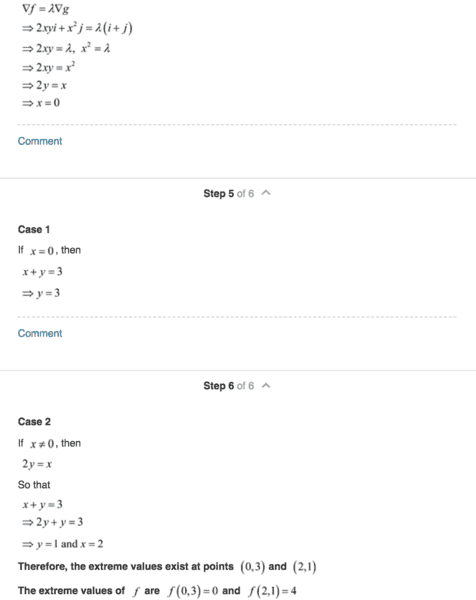# Lagrange multipliers: help solving for x, y and lambda

• rudy
Homework Statement Find the local extreme values of ƒ(x, y) = x2y on the line x + y = 3Homework Equations∇ƒ = λ∇gThe Attempt at a Solution2yxi+x^2j = λi + λj[2yx=λ] [x^2=λ] [x+y=3][2yx=x^2] & [(2y)+y=3][2y

## Homework Statement

Find the local extreme values of ƒ(x, y) = x2y on the line x + y = 3

∇ƒ = λ∇g

## The Attempt at a Solution

2yxi+x^2j = λi + λj
[2yx=λ] [x^2=λ] [x+y=3]
[2yx=x^2] & [(2y)+y=3]
[2y=x] & [y=1]
x=2

This is as far as I got, and gives f(2,1)=4 as the extreme value. The solution I have (chegg.com) lists another possible solution.

On the left column, they give another solution to the equation 2y=x:
They say:
2y=x
x=0
and proceed to use the "x+y=3" equation to give an alternative extrema which is f(0,3)

Can anyone explain how they made the jump from 2y=x to x=0?
Why isn't y=0 another solution? (If x equals and 2y equals x, then y must equal zero)

Thanks in advance, screenshot from solution below

-Rudyrudy said:

## Homework Statement

Find the local extreme values of ƒ(x, y) = x2y on the line x + y = 3

∇ƒ = λ∇g

## The Attempt at a Solution

2yxi+x^2j = λi + λj
[2yx=λ] [x^2=λ] [x+y=3]
[2yx=x^2] & [(2y)+y=3]
[2y=x] & [y=1]
x=2

This is as far as I got, and gives f(2,1)=4 as the extreme value. The solution I have (chegg.com) lists another possible solution.

On the left column, they give another solution to the equation 2y=x:
They say:
2y=x
x=0
and proceed to use the "x+y=3" equation to give an alternative extrema which is f(0,3)

Can anyone explain how they made the jump from 2y=x to x=0?
Why isn't y=0 another solution? (If x equals and 2y equals x, then y must equal zero)

Thanks in advance, screenshot from solution below

-Rudy

View attachment 228274
You ignored the second solution when you reduced ##2xy = x^2## to ##2y=x##. Try writing it as ##2xy-x^2=0## and factoring it.

rudy said:

## Homework Statement

Find the local extreme values of ƒ(x, y) = x2y on the line x + y = 3

∇ƒ = λ∇g

## The Attempt at a Solution

2yxi+x^2j = λi + λj
[2yx=λ] [x^2=λ] [x+y=3]
[2yx=x^2] & [(2y)+y=3]
[2y=x] & [y=1]
x=2

This is as far as I got, and gives f(2,1)=4 as the extreme value. The solution I have (chegg.com) lists another possible solution.

On the left column, they give another solution to the equation 2y=x:
They say:
2y=x
x=0
and proceed to use the "x+y=3" equation to give an alternative extrema which is f(0,3)

Can anyone explain how they made the jump from 2y=x to x=0?
Why isn't y=0 another solution? (If x equals and 2y equals x, then y must equal zero)

Thanks in advance, screenshot from solution below

-Rudy

View attachment 228274

I think their writeup was bad. It should have said "##2xy = x^2 \Rightarrow x = 0 \; {\bf \text{or}} \; 2y = x##."